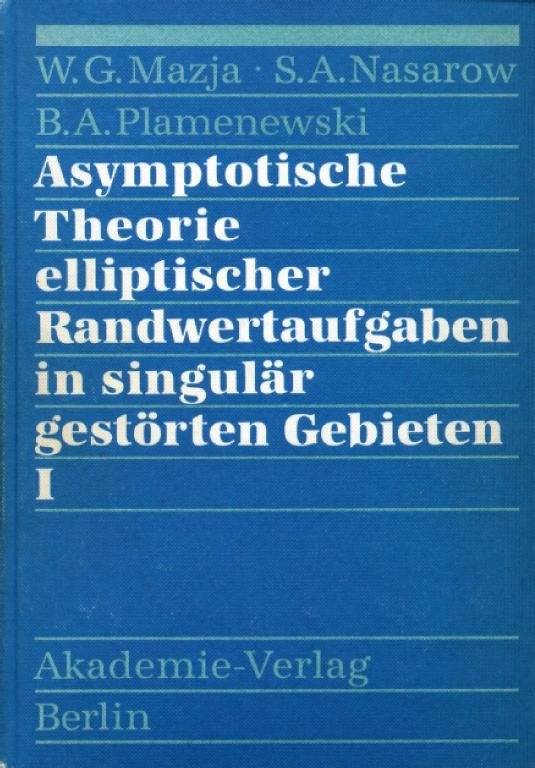# Asymptotische Theorie elliptischer Randwertaufgaben in singulär gestörten Gebieten I

Mazja, W. G.; Nasarow, S. A.; Plamenewski, B. A.,

For the first time, a general and unifying approach to the asymptotic analysis of elliptic boundary value problems in singularly perturbed domains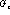is given. For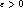, theare smoothly bounded domains which converge, iftends to zero, towards a domain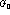with irregular boundary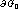. In the present volume,contains a finite set of corners, conical or isolated points:arises out ofby rounding off corners [resp. conical points] or by removing subsets of diameter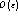--"small holes". The general problem posed consists in the construction of asymptotic expansions, in powers of, for the solutions of boundary value problems in, for, and in the proof of their convergence.

The principles of the procedures used are first demonstrated in the cases of model problems: Dirichlet and Neumann problems for the Poisson and Helmholtz equations in a three-dimensional bounded domain with a small hole at the origin, i.e.,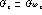,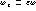, G andsmoothly bounded domains containing the origin 0, and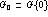. In this, two possibilities of construction are proposed as follows. (1) "Matched expansions": General asymptotic expansions with spherical harmonics are set up (a) for the solution of the boundary value problem in, with respect to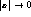, and (b) for the solution in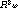, vanishing at infinity, with respect to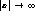; the coefficients of the expansions will then follow from comparison within an intermediate region where both expansions are valid. (This method has been used, in principle, by A. M. Ilin [Mat. Sb. (N.S.) 99 (141) (1976), no. 4, 514-537; MR 53 #11214].) (2) "Compound expansions": Here the coefficient functions of the-powers (no integers, in general) are, alternately, solutions of two fixed "limit problems"--the inner problem in G (without hole), and the outer problem in; the boundary conditions of each problem are to compensate, up to terms of major order in, the defect of the boundary values imposed by the solution of the preceding one (in the other domain). Moreover, in the case of nonhomogeneous elliptic operators, usually "exchanges of defects" must, additionally, be brought about in order to replace defects of the boundary values by those of the right-hand sides. Nevertheless, the second method proves more transparent and highly universal; it is, therefore, almost exclusively used throughout the book. (It might be considered a modification of the method of coupled iteration processes used for asymptotic expansions for solutions of singularly perturbed differential equations, by M. I. Vishik and L. A. Lyusternik [Uspekhi Mat. Nauk (N.S.) 12 (1957), no. 5(77), 3-122; MR 20 #2539].)

For the same model problems, but in domains with-smoothed corners [resp. conical points], the authors show that the method, in principle, will work in the same way.

The theoretical kernel of the book is contained in Section 4 of Chapter II: For general boundary value problems in domains, complete asymptotic expansions with powers of the small parameterare constructed by algorithm, and their convergence is proved in appropriate spaces--and this uniformly for all the cases of irregularities considered. Here the general theory on the solvability of elliptic problems in domains with nonsmooth boundaries, developed during the last twenty years, has been crucial.

In this book, considerable attention is devoted above all to concrete problems of mathematical physics, especially elasticity theory; asymptotics of stress intensity factors, energy integrals, and eigenvalues are studied in detail. The book is, to a great extent, based on results of the authors themselves. Volume II is announced with corresponding asymptotic problems for domains with edges, for "thin" domains, or domains with narrow channels, for equations of higher order, for equations with rapidly oscillating coefficients, etc.

Reviewed by Dietrich Gohde